Courses

# Test: Enthalpy Of Combustion

## 17 Questions MCQ Test Chemistry for JEE | Test: Enthalpy Of Combustion

Description
This mock test of Test: Enthalpy Of Combustion for JEE helps you for every JEE entrance exam. This contains 17 Multiple Choice Questions for JEE Test: Enthalpy Of Combustion (mcq) to study with solutions a complete question bank. The solved questions answers in this Test: Enthalpy Of Combustion quiz give you a good mix of easy questions and tough questions. JEE students definitely take this Test: Enthalpy Of Combustion exercise for a better result in the exam. You can find other Test: Enthalpy Of Combustion extra questions, long questions & short questions for JEE on EduRev as well by searching above.
QUESTION: 1

### Direction (Q. Nos. 1-11) This section contains 11 multiple choice questions. Each question has four choices (a), (b), (c) and (d), out of which ONLY ONE option is correct. Q. Calorific value of H2 is - 143 kJ g-1.Thus, ΔfH° of H2O is

Solution:

Calorific value is given for 1 gram and enthalpy of formation is defined for one mole of substance formed so for one mole of water formed we need two gram of hydrogen so the enthalpy of formation of H2O will be 2×Calorific value. Or 2×(-143) = -286 kJ/ mol

QUESTION: 2

### In the complete combustion of C2H6, 54 g of H2O is formed and 370 kcal of heat is evolved. Thus, ΔCH° of C2H6 is

Solution:

54 gram of water is formed or three moles of water is formed.
C2H6 + (7/2)O2 → 2CO2 + 3H2O
So it indicates that only one mole of C2H6 is used in the combustion so the heat released is itself the value of heat of combustion of Ethane.

QUESTION: 3

### The enthalpy of combustion of methane, graphite and dihydrogen at 298 K are - 890.3 kJ mol-1, - 393.5 kJ mol-1 and - 285.8 kJ mol-1 respectively. Enthalpy of formation of CH4(g) will be

Solution: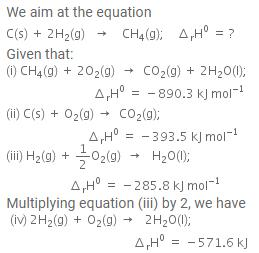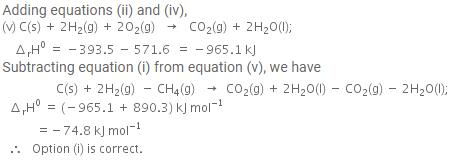QUESTION: 4

The reaction of cyanamide, NH2CN (s) was carried out in a bomb calorimeter, and ΔU was found to be - 742.7 kJ mol-1 at 298 K. Thus, ΔrH° at 298 K for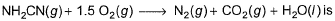Solution:

We know, from first law of thermodynamics,
enthalpy change{∆H} = ∆U{internal energy} + ∆ngRT
Where,
∆ng = no of mole of gaseous products - no of mole of gaseous reactant
So the reaction follows :
NH2CN(s) +(3/2)O2(g) → N2(g)+CO2(g)
∆ng = np - nr
= (1 + 1) - 3/2 = 1/2 mol
= 0.5 mol
Now, ∆H = ∆U + ∆ngRT
= -742.7 KJ +(0.5mol × 8.314 J/mol/K × 298K)
= -742 KJ + 1238.786 J
= -742 KJ + 1.238786 KJ
= -741.46 KJ
Hence, enthalpy change for the reaction is 741.46 KJ for combustion of 1 mole of NH2CN.

QUESTION: 5

Based on the following thermochemical reactions at 298 K and 1 bar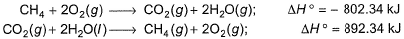Q. Enthalpy of vaporisation of H2O (l) is

Solution:

Enthalpy of vaporization is defined for a substance when one mole of the substance is converted into the gaseous state. To obtain the enthalpy of vaporization of H2O liquid we will add reaction 1 and reaction 2 and then divide the enthalpy of x to since we need to calculate the enthalpy of vaporization for single mole of H2O

QUESTION: 6

For the following combustion reaction of benzoic acid at 298 K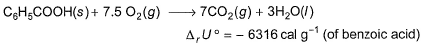Q. Thus enthalpy of combustion ΔCH° at 298 K is

Solution:
QUESTION: 7

The standard enthalpies of formation of CO2(g), H2O (l) and glucose (s) at 25°C are - 400 kJ mol-1, - 300 kJ mol-1 and - 1300 kJ mol-1 respectively. The standard enthalpy of combustion per gram of glucose at 25°C is

[JEE Advanced 2013]

Solution: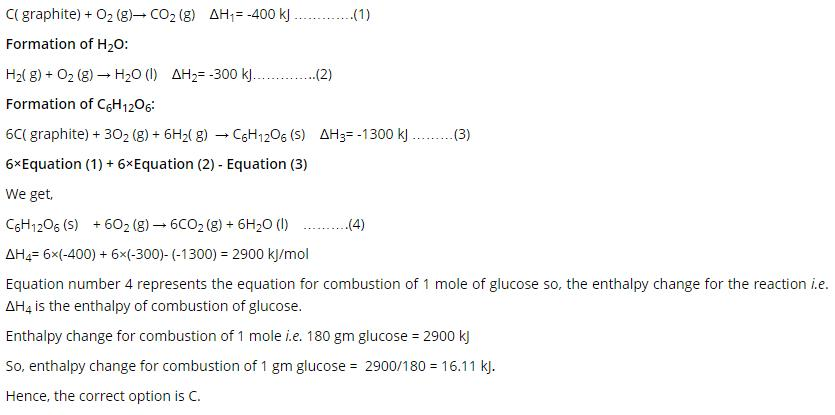QUESTION: 8

For the complete combustion of ethanol,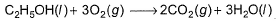the amount of heat produced as measured in bomb calorimeter is 1364.47 kJ mol-1 at 25°C. Assuming ideality, the enthalpy of combustion, ΔCH° for the reaction Will be (R = 8.314 JK-1 mol-1)

[JEE Main 2014]

Solution:

∆H = ∆E + ∆ngRT
∆E = -1364.47 kJ mol-1 and T = 298 K
∆ng = 2-3 = -1
∆H = -1364.47 -1(8.314)289/1000 = -1366.95 kJ mol-1

QUESTION: 9

Given,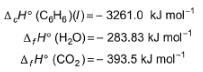Q. Thus, ΔfH° of (C6H6) (l) is

Solution:

C6H6(l) + 15/2O2(g) → 6CO2(g) + 3H2O(l)
∆Hr = (∆Hc)C6H6 ⇒-3261  = ∑Hproduct - ∑Hreactant = 6(-393.5) -3(-283.83) - ∆fHC6H6
∆Hf = 48 kJ mol-1

QUESTION: 10

ΔfU° of formation of CH4(g) at a certain temperature is - 393 kJ mol-1. The value of ΔfH° is

Solution:

The balanced equation for combustion of methane is:
CH4(g)+2O2(g)→CO2(g)+2H2O(l)Here, Δng=1−3=−2
ΔH0=ΔU0+ΔngRT
ΔH=−393−2RT
∴ΔH0<ΔU0

QUESTION: 11

Heat of combustion of H2(g) is - 58 kcal mol-1 at 298 K and constant pressure.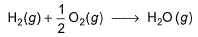Q. Temperature of a hydrogen-oxygen flame if H2(g) is used as fuel is

Solution:
QUESTION: 12

Direction (Q. Nos. 12-15) This section contains a paragraph, wach describing  theory, experiments, data etc. three Questions related to paragraph have been  given.Each question have only one correct answer among the four given  ptions  (a),(b),(c),(d).

Passage I

Octane (C8H18), a primary constituent of gasoline, burns in air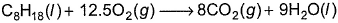A 1.00 g sample of octane is burned in a constant volume calorimeter containing 1.20 kg of water. The temperature of water and the bomb rises by 8.2 K. The heat capacity of the bomb is 837.0 JK-1. Molar heat capacity of water is 4.184 J g-1 K-1. Initial temperature is 298 K.

Q. ΔCU° (per mol of C8H18) is

Solution: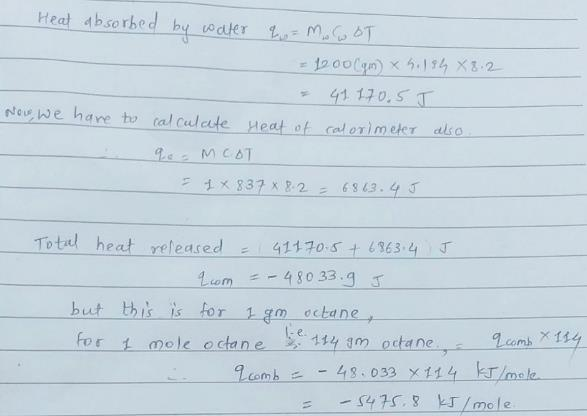QUESTION: 13

Passage I

Octane (C8H18), a primary constituent of gasoline, burns in airA 1.00 g sample of octane is burned in a constant volume calorimeter containing 1.20 kg of water. The temperature of water and the bomb rises by 8.2 K. The heat capacity of the bomb is 837.0 JK-1. Molar heat capacity of water is 4.184 J g-1 K-1. Initial temperature is 298 K.

Q. ΔCH° (per mol of C8H18) is

Solution:
QUESTION: 14

Passage II

Heat of combustion of sucrose under standard state is - 5640 kJ mol-1. A healthy person takes 20 breaths per minute, and each breath consumer 480 mL of air. Inhaled and exhaled air contains 20% and 15% of oxygen by volume.

Q. If oxygen retained in the body is used in the combustion of sucrose, number of moles of sucrose combusted per day is

Solution:
QUESTION: 15

Passage II

Heat of combustion of sucrose under standard state is - 5640 kJ mol-1. A healthy person takes 20 breaths per minute, and each breath consumer 480 mL of air. Inhaled and exhaled air contains 20% and 15% of oxygen by volume.

Q. Heat evolved as a result of combustion of sucrose is

Solution:
*Answer can only contain numeric values
QUESTION: 16

Direction (Q. Nos. 16 and 17) This section contains 2 questions. when worked out will result in an integer from 0 to 9 (both inclusive).

Q. Given, ΔfH° of the following in kJ mol-1.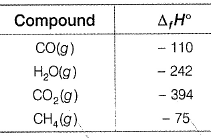Determine the ratio taking derived values per unit volume of fuel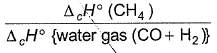Solution:
*Answer can only contain numeric values
QUESTION: 17

ΔCH° (cyclopropane) = - 2091.97 kJ mol-1
ΔCH° (propene) = - 2058.32 kJ mol-1

Q. What is ΔrH° (in kcal) propenecyclopropane?

Solution:

Cyclopropane + O2 → CO2(g) + H2O(l)  ΔCH°(cyclopropane) = -2091.97 kJmol-1 -----(i)
Propene + O2 → CO2(g) + H2O(l)  ΔCH°(propene) = -2058.32 kJmol-1 -----(ii)
On (ii)-(i), we get
Propene → Cyclopropane     ∆H = 33.65 kJ ml-1
1 cal = 4.18 J
So, we have 33.65/4.18 = 8 kcal